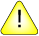This version of this document is no longer maintained. For the latest documentation, see http://www.qnx.com/developers/docs.

# expr

Evaluate arguments as an expression (POSIX)

## Syntax:

`expr operand...`

## Options:

operand
Operand expression to evaluate.

## Description:

The expr utility evaluates a single expression and writes the result to the standard output. The expression is formed from integer and string symbols in combination with the following operators:

`(  )  |  &  =  >  >=  <  <=  !=  +  -  *  /  %  :`

In the following table, expressions are listed in order of decreasing precedence, with equal-precedence operators grouped together. All of the operators are left-associative. Note that a string operand is an argument that can't be identified as an integer argument or as one of the above operators.

Expression Description
integer An argument consisting only of an (optional) unary minus followed by digits
string A string argument
( expr ) Grouping symbols; you can place any expression inside the parentheses.
expr1 : expr2 Matching expression
expr1 * expr2 Integer multiplication
expr1 / expr2 Integer division, producing an integer result
expr1 % expr2 Remainder of integer division
expr1 - expr2 Integer subtraction
expr1 = expr2 Equal*
expr1 > expr2 Greater than*
expr1 >= expr2 Greater than or equal*
expr1 < expr2 Less than*
expr1 <= expr2 Less than or equal*
expr1 != expr2 Not equal*
expr1 & expr2 Returns the evaluation of expr1 if neither expression evaluates to null or zero; otherwise, returns zero
expr1 | expr2 Returns the evaluation of expr1 if it's neither null nor zero; otherwise returns the evaluation of expr2

* Returns the result of a decimal integer comparison if both arguments are integers; otherwise, returns the result of a string comparison. The result of each comparison is 1 if the specified relation is TRUE or 0 if FALSE.

The ":" matching operator compares the string resulting from the evaluation of expr1 with the regular expression pattern resulting from the evaluation of expr2. Usually, this operator returns the string representing the number of characters matched ("0" on failure). However, if the pattern contains at least one regular expression subexpression \(...\), the string corresponding to \1 is returned (see grep).

## Examples:

`expr 1 + 1`

Logical 1 or 0 :

`expr 1 \| 0`

Logical 1 and 0 :

`expr 1 \& 0`

`expr \$(expr \$a + 1)`

Count the characters in \$var :

`expr "\$var" : '.*'`

Compare \$a to a possible =

`expr x\$a = x=`

## Exit status:

0
The expression evaluates to neither null nor zero.
1
The expression evaluates to null or zero.
2
Invalid expressions were found.
>2
An error occurred.

## Caveats:

The syntax for expr requires special attention. Many of the operators are also shell control operators or reserved words, so they have to be escaped on the command line. In addition, each part of the expression is composed of separate arguments, so you should use blanks liberally. For example:

Instead of entering: You should enter:
expr "1 + 2" expr 1 + 2
expr 1 + (2 * 3) expr 1 + \( 2 \* 3 \)
expr 1+2 expr 1 + 2

In many cases, the arithmetic and string features provided as part of the shell command language are easier to use than their equivalents in expr. However, you may need expr to run older UNIX shell scripts.

When you want to specify the filesystem root, avoid the / character as a standalone argument -- expr interprets it as the division operator. Use // instead.